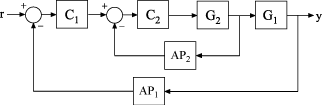# getPoints

Get list of analysis points in generalized model of control system

## Syntax

``points = getPoints(T)``

## Description

example

````points = getPoints(T)` returns the names of all analysis-point locations in a generalized state-space model of a control system. Use this function to query the list of available analysis points in the model for control system analysis or tuning. You can refer to the analysis-point locations by name to create design goals control system tuning or to compute open-loop and closed-loop responses using analysis commands such as `getLoopTransfer` and `getIOTransfer`.```

## Examples

collapse all

Build a closed-loop model of a cascaded feedback loop system, and get a list of analysis point locations in the model.

Create a model of the following cascaded feedback loop. ${C}_{1}$ and ${C}_{2}$ are tunable controllers. $A{P}_{1}$ and $A{P}_{2}$ are points of interest for analysis, which you mark with `AnalysisPoint` blocks.```G1 = tf(10,[1 10]); G2 = tf([1 2],[1 0.2 10]); C1 = tunablePID('C','pi'); C2 = tunableGain('G',1); AP1 = AnalysisPoint('AP1'); AP2 = AnalysisPoint('AP2'); T = feedback(G1*feedback(G2*C2,AP2)*C1,AP1);```

`T` is a `genss` model whose Control Design Blocks include the tunable controllers and the switches `AP1` and `AP2`.

Get a list of the loop-opening sites in `T`.

`points = getPoints(T)`
```points = 2x1 cell {'AP1'} {'AP2'} ```

`getPoints` returns a cell array listing loop-opening sites in the model.

For more complicated closed-loop models, you can use `getPoints` to keep track of a larger number of analysis points.

## Input Arguments

collapse all

Model of a control system, specified as a generalized state-space (`genss`) model. Locations in the model at which you can calculate system responses or specify design goals for tuning are marked by `AnalysisPoint` blocks in `T`.

## Output Arguments

collapse all

Analysis-point locations in the control system model, returned as a cell array of character vectors. This output is obtained by concatenating the `Location` properties of all `AnalysisPoint` blocks in the control system model.

## Version History

Introduced in R2014b